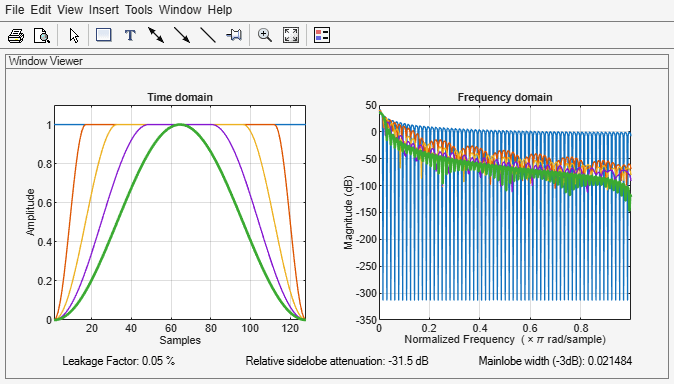# tukeywin

Tukey (tapered cosine) window

## Syntax

``w = tukeywin(L,r)``

## Description

example

````w = tukeywin(L,r)` returns an `L`-point Tukey window with cosine fraction `r`.```

## Examples

collapse all

Compute 128-point Tukey windows with five different values of `r`, or "tapers." Display the results using `wvtool`.

```L = 128; t0 = tukeywin(L,0); % Equivalent to a rectangular window t25 = tukeywin(L,0.25); t5 = tukeywin(L); % r = 0.5 t75 = tukeywin(L,0.75); t1 = tukeywin(L,1); % Equivalent to a Hann window wvtool(t0,t25,t5,t75,t1)```## Input Arguments

collapse all

Window length, specified as a positive integer.

Data Types: `single` | `double`

Cosine fraction, specified as a real scalar. The Tukey window is a rectangular window with the first and last `r``/2` percent of the samples equal to parts of a cosine. For example, setting `r` `= 0.5` produces a Tukey window where `1/2` of the entire window length consists of segments of a phase-shifted cosine with period `2``r` `= 1`. If you specify `r` `≤ 0`, an `L`-point rectangular window is returned. If you specify `r` `≥ 1`, an `L`-point von Hann window is returned.

Data Types: `single` | `double`

## Output Arguments

collapse all

Tukey window, returned as a column vector.

## Algorithms

The following equation defines the L-point Tukey window:

`$w\left(x\right)=\left\{\begin{array}{ll}\frac{1}{2}\left\{1+\mathrm{cos}\left(\frac{2\pi }{r}\left[x-r/2\right]\right)\right\},\hfill & 0\le x<\frac{r}{2}\hfill \\ 1,\hfill & \frac{r}{2}\le x<1-\frac{r}{2}\hfill \\ \frac{1}{2}\left\{1+\mathrm{cos}\left(\frac{2\pi }{r}\left[x-1+r/2\right]\right)\right\},\hfill & 1-\frac{r}{2}\le x\le 1\hfill \end{array}$`

where x is an L-point linearly spaced vector generated using `linspace`. The parameter r is the ratio of cosine-tapered section length to the entire window length with 0 ≤ r ≤ 1. For example, setting r = 0.5 produces a Tukey window where 1/2 of the entire window length consists of segments of a phase-shifted cosine with period 2r = 1. If you specify r ≤ 0, an L-point rectangular window is returned. If you specify r ≥ 1, an L-point von Hann window is returned.

 Bloomfield, P. Fourier Analysis of Time Series: An Introduction. New York: Wiley-Interscience, 2000.